• Partition 246 and 173 into hundreds, tens and units before adding these values separately.
• Start by adding the units (ones) in the rightmost column.
• 6 + 3 = 9 units.
• In the tens column, 4 + 7 = 11 tens. 11 has two digits.
• We can only write one digit per column.
• We can regroup 10 tens to make a hundred, which can be carried over to the hundreds column.
• This leaves 1 group of ten behind in the tens column.
• In the hundreds column we have 2 + 1 + the extra 1 which was carried from the tens column.
• In total there are 4 groups of hundred in the hundreds column.
• We can now read our answer: 246 + 173 = 419.

A group of 10 units (ones) can be regrouped to make 1 ten and a group of 10 tens can be regrouped to make 1 hundred.

We only write one digit per column and carry over the tens digit if necessary.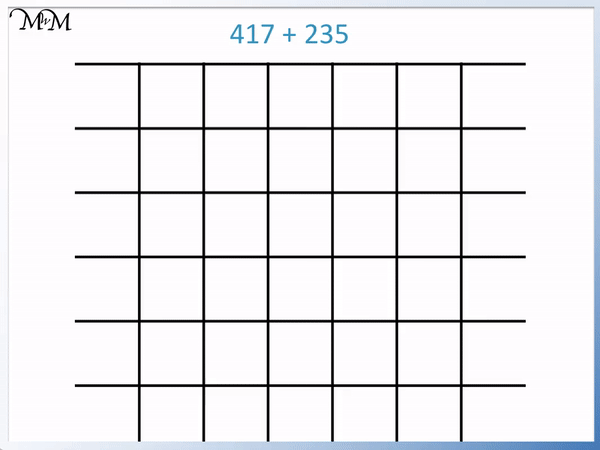• Set out the column addition of 417 + 235 by lining up the hundreds, tens and units digits of each number.
• Adding the digits from right to left, starting with the units column, we have:
• 7 + 5 = 12.
• 12 contains two digits and so, we write down '2' in the units column and carry the '1' into the tens column.
• Adding the digits in the tens column, 1 + 3 + the 1 we carried equals 5.
• Adding the digits in the hundreds column, 4 + 2 = 6.
• 417 + 235 = 652.#### 3-Digit Addition with Regrouping Twice#### Column Addition with Regrouping: Interactive Questions# 3-Digit Column Addition with Regrouping

In this lesson we use the column addition method to add 3-digit numbers.

To add numbers using the column addition method, line up the digits of each number above each other and add them separately. The sum of each digit is written below, with one digit per box.

We use the method of regrouping when more than one digit is produced after adding the digits in a place value column.

In maths, regrouping is the process of moving values between place value columns. When carrying out an addition, regrouping is often used to move a group of ten to the next place value column up. Some examples of regrouping are replacing 10 ones with 1 group of ten, or 10 tens with 1 group of one hundred.

When teaching addition, some teachers use the word 'carrying' as well as the word 'regrouping'.

Carrying and regrouping mean the same in addition. Carrying is the movement of a ten over to the next place value column. Regrouping is also used in subtraction, where it is sometimes known as borrowing.

We will learn about regrouping in this lesson by considering some examples of addition involving regrouping.

Here is an example of 246 + 173: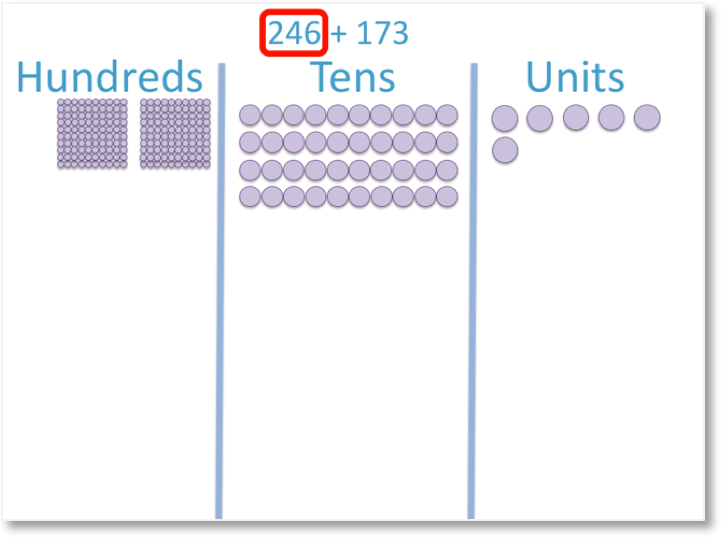The number 246 has 6 units (or ones). These are shown by the six purple counters in the units column.

It has 4 tens. These are shown by the four groups of ten purple counters in the tens column.

It also has >2 hundreds, which are shown by the two groups of one hundred purple counters in the hundreds column.The number 173 has 3 units, which are shown by the three green counters in the units column.

It has 7 tens. These are shown by the seven groups of ten green counters in the tens column.

It also has 1 hundred. This is shown by the one group of one hundred green counters in the hundreds column.

Now that we have lined up the digits of each number in their place value columns, we can add the digits in each column.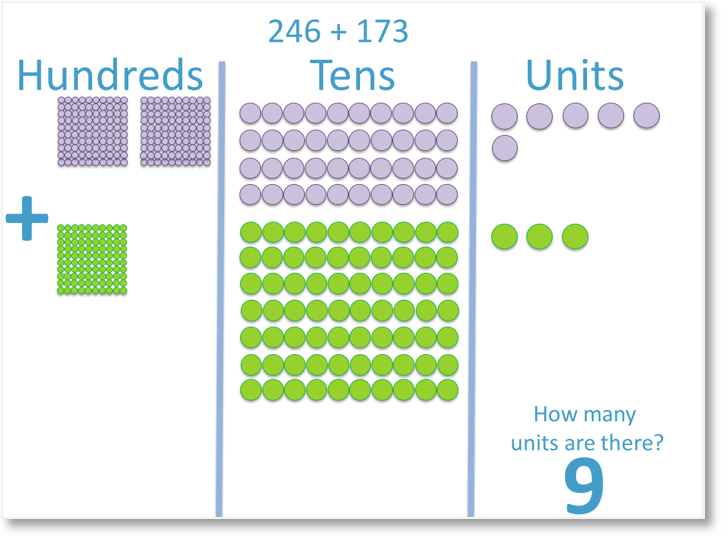We have a total of 9 counters in the units column.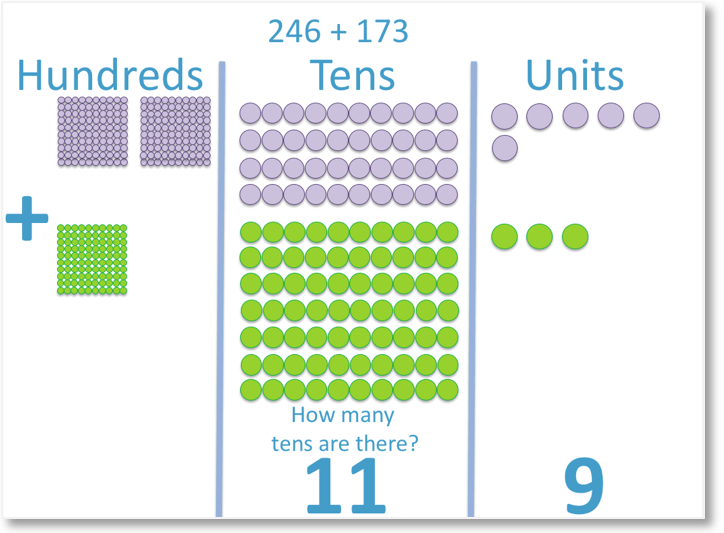Next, we look at the tens column. We have a total of 11 tens.

The number 11 has two digits.

We can’t have more than one digit in each place value column.

We therefore need to regroup 100 counters to carry over to the hundreds column.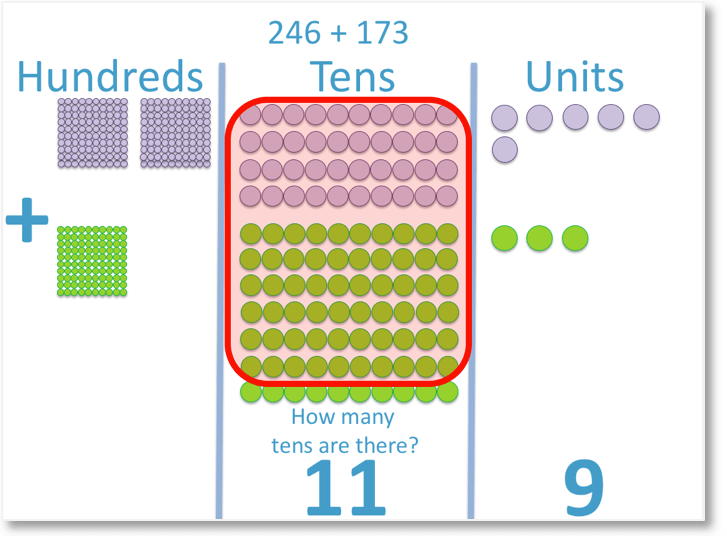In the hundreds column, we can have groups of one hundred. Therefore, we can regroup one hundred counters from the tens column and carry them over to the hundreds column.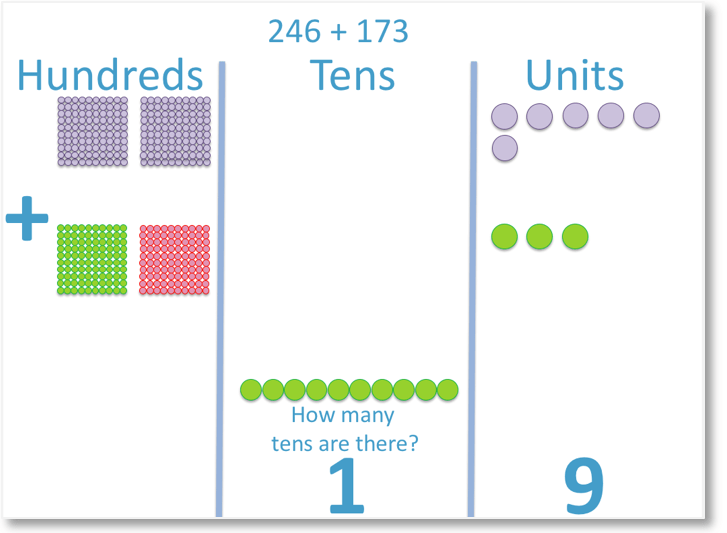Now that we have carried 1 hundred over to the hundreds column, we are left with 1 ten in the tens column.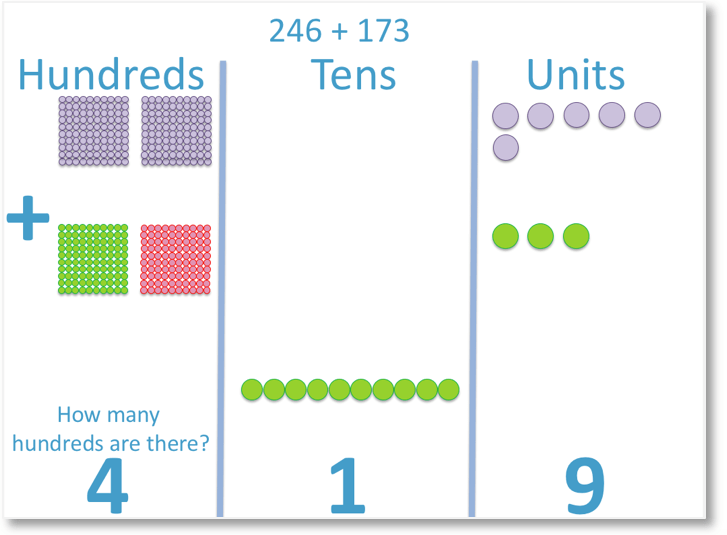Finally, we look at the hundreds column. We have 4 groups of one hundred counters.

Therefore,

246 + 173 = 419

When teaching regrouping, it is useful to introduce the concept visually as above. You can use counters or Dienes blocks to help teach this. Once the idea of regrouping is understood, column addition is best taught methodically with written digits.We use regrouping whenever the sum of the digits in each column of the column addition is larger than 9. We regroup ten ones to make 1 group of ten or we can regroup ten groups of ten to make one group of one hundred.

When using column addition, the regrouping process is often called carrying as the extra digit is carried across to be added with the next column of digits.

We will look at this example again, but this time we will use numbers to represent each digit and set out the calculation using the column addition method.We line up the digits of each number according to their place values.

We begin by adding the digits in the units column.6 + 3 = 9

We write the 9 in the units column, underneath the 6 and the 3.

Next, we add the digits in the tens column.4 + 7 = 11

The number 11 has 1 unit, which we keep in the tens column, and 1 ten, which we carry over to the hundreds column and write below the line.

Finally, we add the digits in the hundreds column. We must also remember to add the 1 that we carried over.2 + 1 + 1 = 4

Therefore,

246 + 173 = 419We will look at some further examples of column addition involving regrouping.

Here we have the 3-digit numbers 417 + 235.

We set the numbers out with the column addition method, with one digit per box and one number above the other.

We will add the units first.

7 + 5 = 12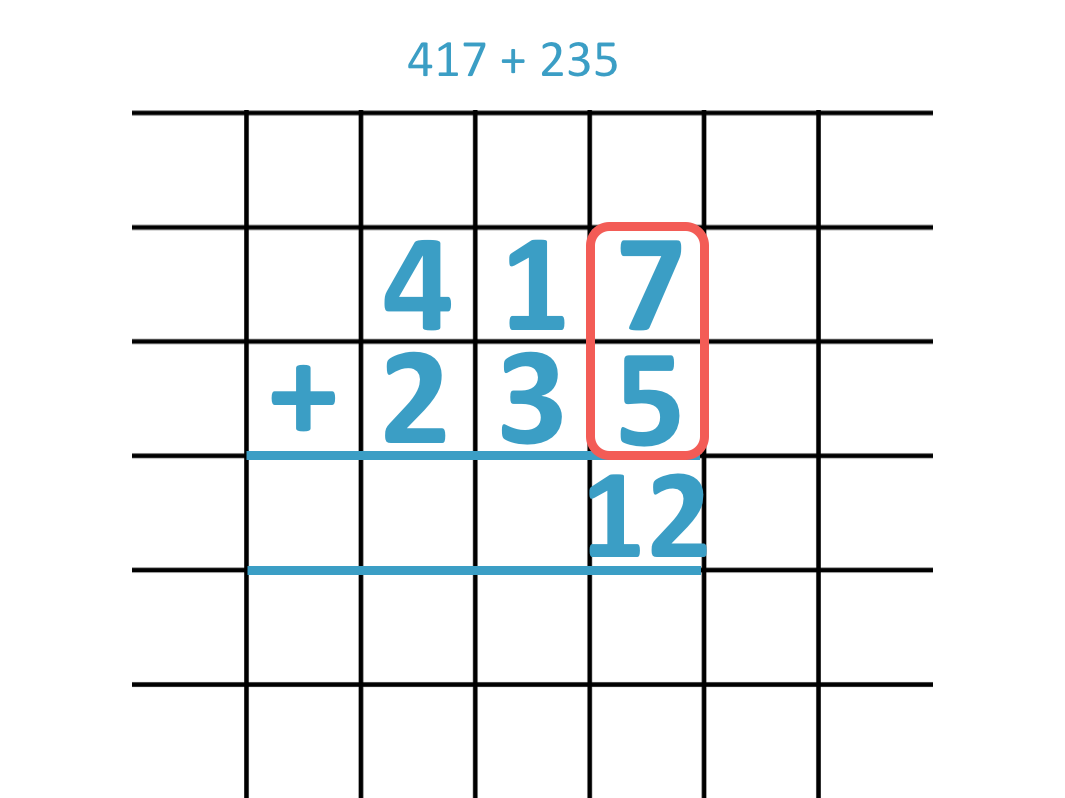We cannot have two digits written in one box or one place value column and so, we know we need to regroup.

We write down the units digit of '12', which is '2'.

The '1' ten is carried over to the tens column. We do not write it between the lines. Instead we can write it below the line.We need to add this '1' that has been carried in the tens column.

1 + 3 + the '1' which was carried = 5Adding the digits in the hundreds column we have 4 + 2 = 6.In this example of adding three-digit numbers we have 863 + 524.

We set the numbers out in the column addition method with the first number above the second.

We can add the units column digits easily since 3 + 4 = 7.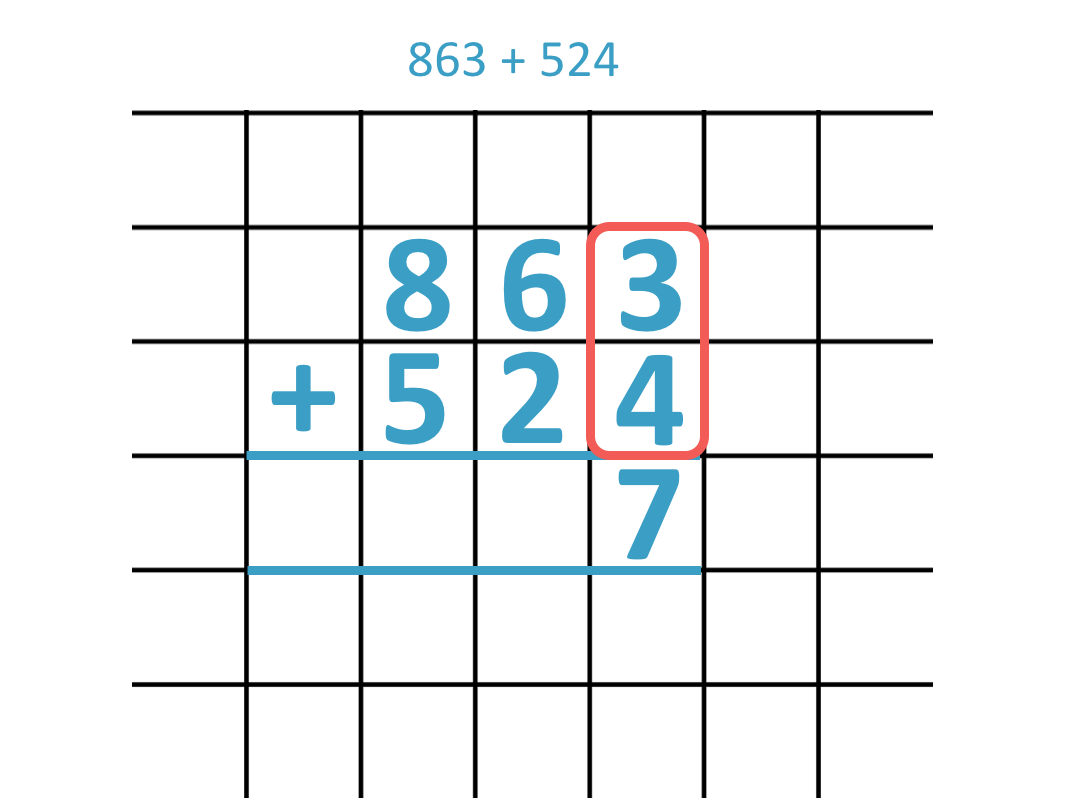In the tens column we have 6 + 2 = 8.Adding the hundreds digits we have 8 + 5 = 13.13 contains two digits and so, we must carry the '1' into the next column.

Ten hundreds make 1 thousand.

We write the '3' in the hundreds column and the '1' is carried into the thousands column on the left.#### 3-Digit Column Addition with Regrouping Twice

Once the regrouping process has been taught, it can be applied multiple times within the same question. Some 3-digit addition problems will involve regrouping twice within one sum.

Here is the example of 365 + 187.Starting with 5 + 7 in the units column, we get 12.

12 contains two digits and so we carry the '1' over to the tens column.

We then add the tens column digits.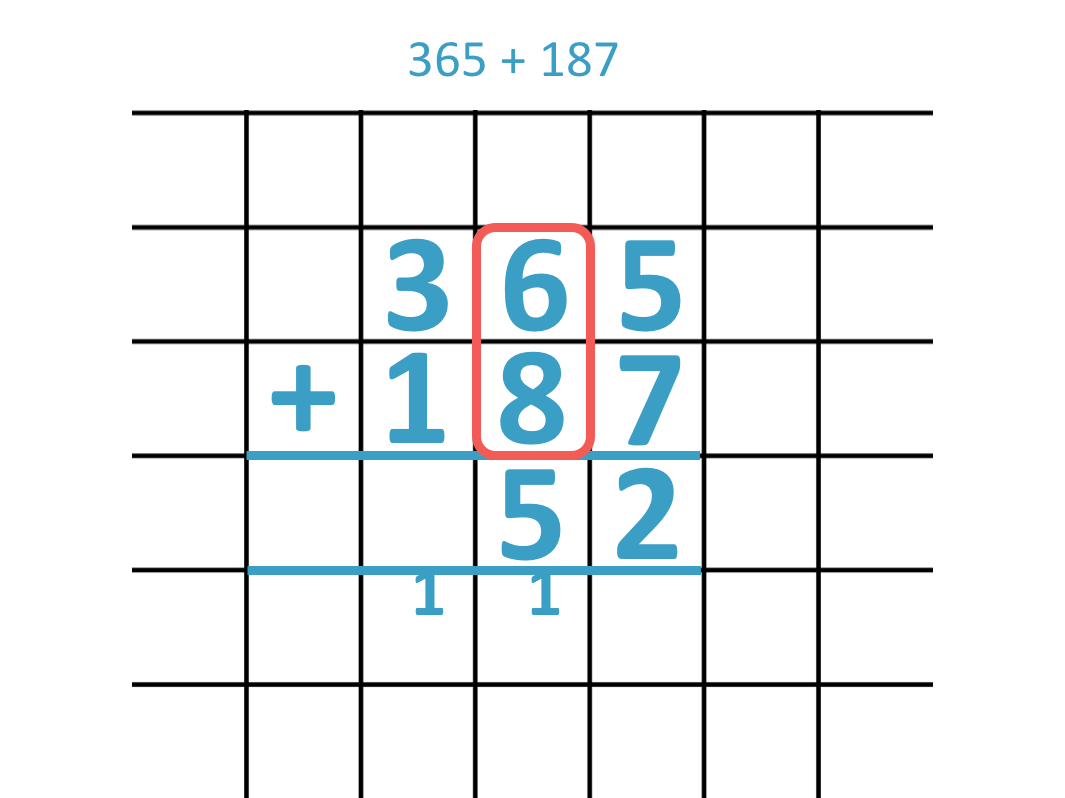Adding the digits in the tens column, we have 6 + 8 from the two numbers given, plus the '1' that we carried during the regrouping process.

6 + 8 + 1 = 15.

Again 15 contains two digits, so we need to regroup.

We carry the '1' over from the tens to the hundreds column, leaving the '5' in the tens column.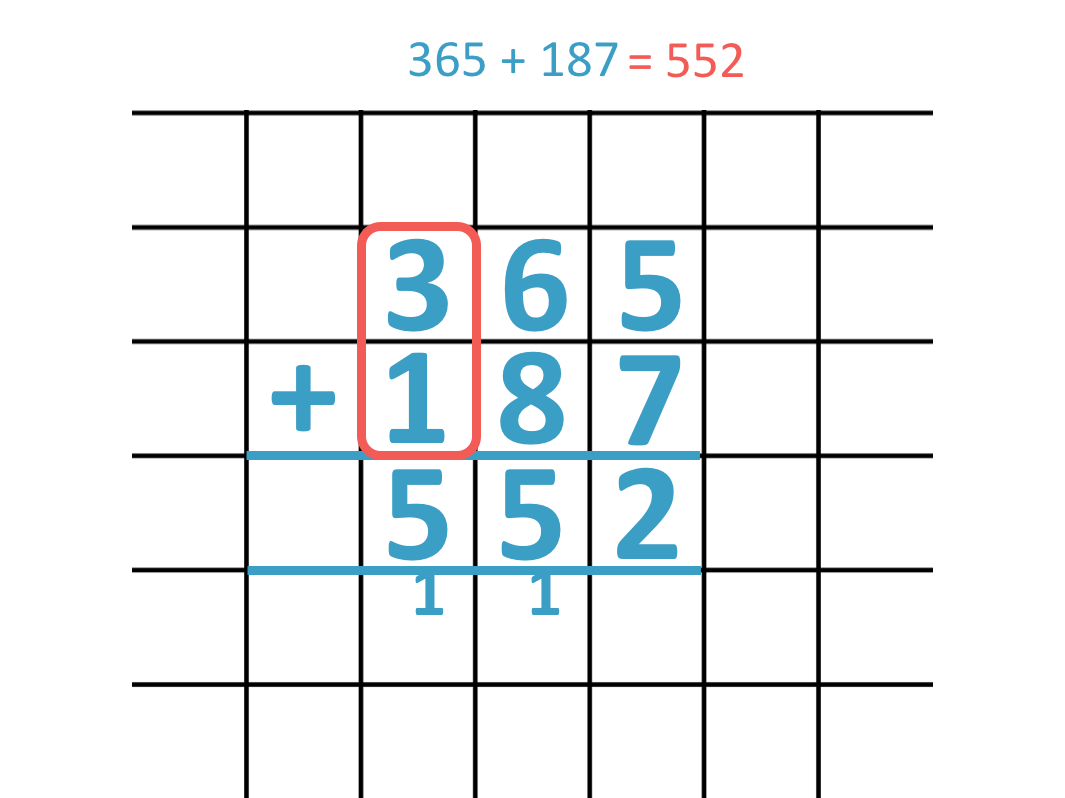Finally, we add the hundreds column digits. We have 3 + 1 in the two numbers given and we need to add the '1' that was carried.

3 + 1 + 1 = 5.

We have 365 + 187 = 552.

We carried twice in this sum, regrouping from the ones column to the tens column and also from the tens column to the hundreds column.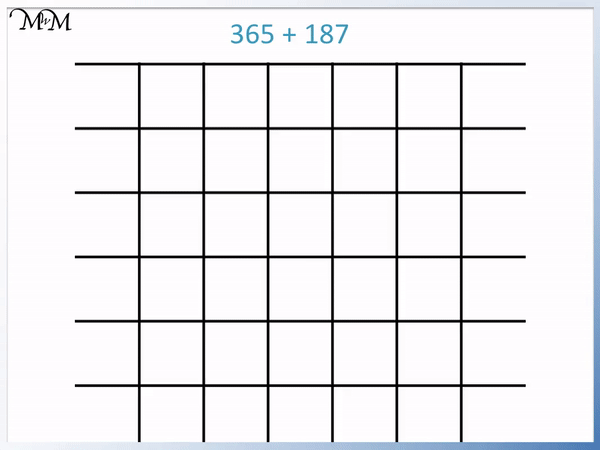Here is another example with regrouping twice.

We are adding the 3-digit numbers 728 + 644.

We set the digits out above each other as per the column addition method and add the digits in the units (ones) column.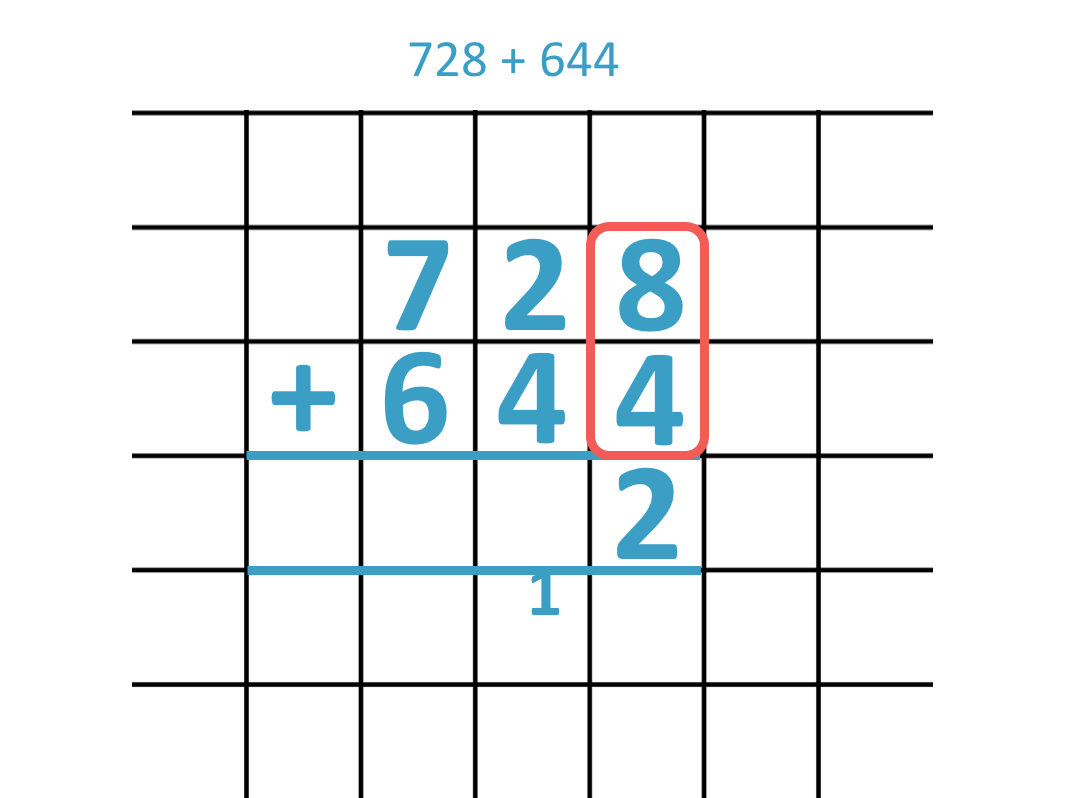8 + 4 = 12, which is a two-digit number. We need to carry the '1' across into the tens column, leaving the '2' in the units column.

Now we add the tens column digits, plus the '1' that was carried.

In the tens column we have, 2 + 4 plus the one that we carried.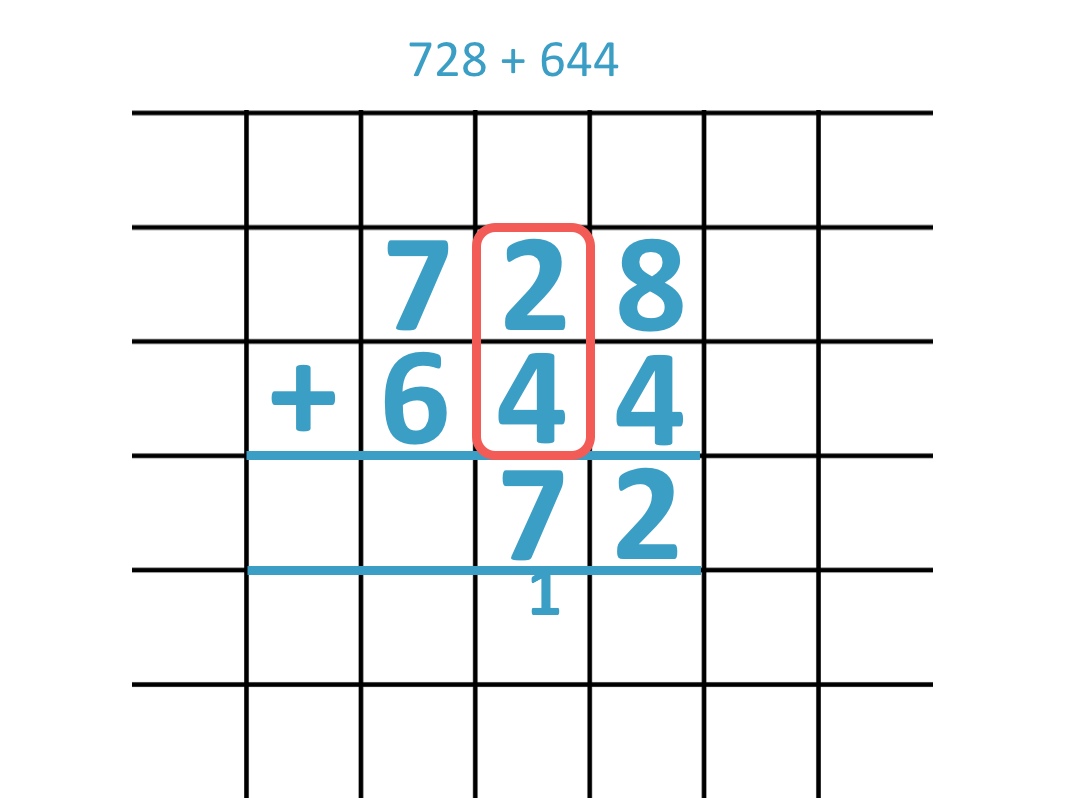2 + 4 + 1 = 7, which only contains one digit. We can simply write '7' in the tens column.

Now we add the hundreds digits.

We have 7 + 6, which equals 13.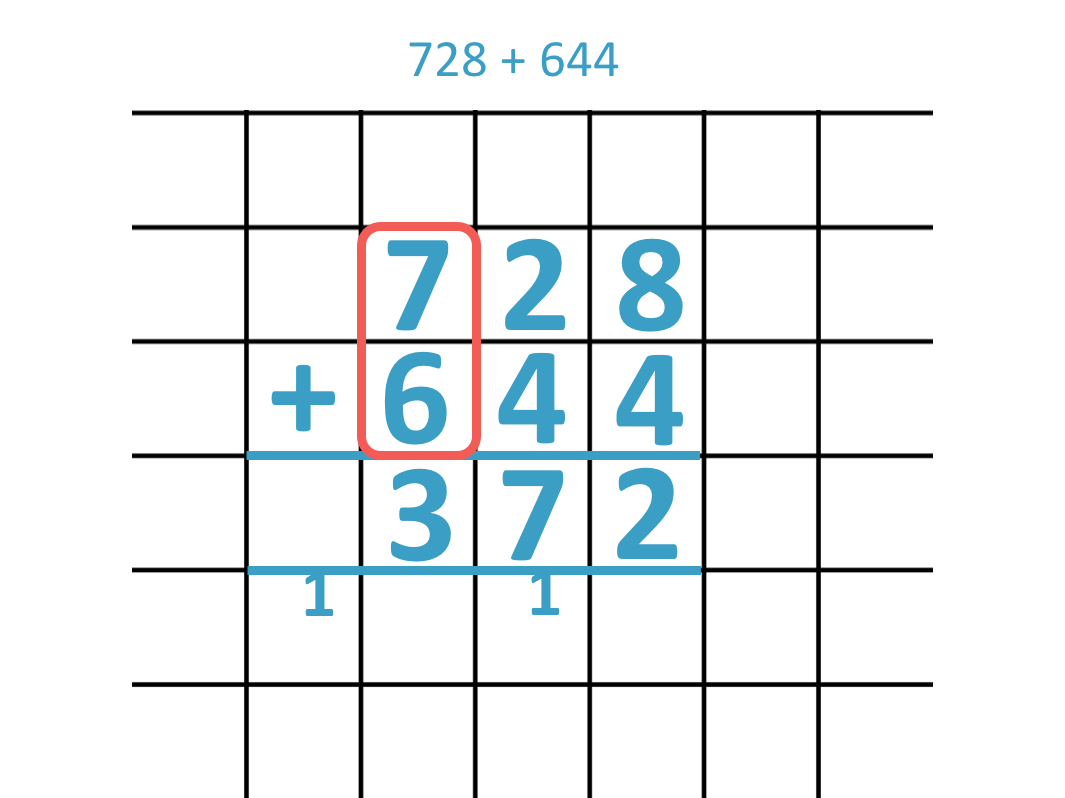We cannot write '13' in the hundreds column alone because it contains 2-digits.

We carry the '1' into the thousands column.

We can regroup ten hundreds to make one thousand.Since there is no other digit in the thousands column, we simply write the '1' here.

We have added two 3-digit numbers to make a 4-digit number because we regrouped from the hundreds column into the thousands column.Here is another example of adding two 3-digit numbers to make a 4-digit number in the thousands.

We have 876 + 552.

Adding the units column, we have 6 + 2, which equals 8.

There is no need to regroup.We move onto the tens column. 7 + 5 = 12 and so, we regroup the 10 tens to make '1' hundred.

We write the '1' below the hundreds column.Now adding the hundreds column we have 8 + 5 plus the '1' that we carried from the tens column.

8 + 5 + 1 = 14.

We need to regroup these hundreds into a thousand.

We carry the '1' over into the thousands column and leave the '4' hundreds behind in the hundreds column.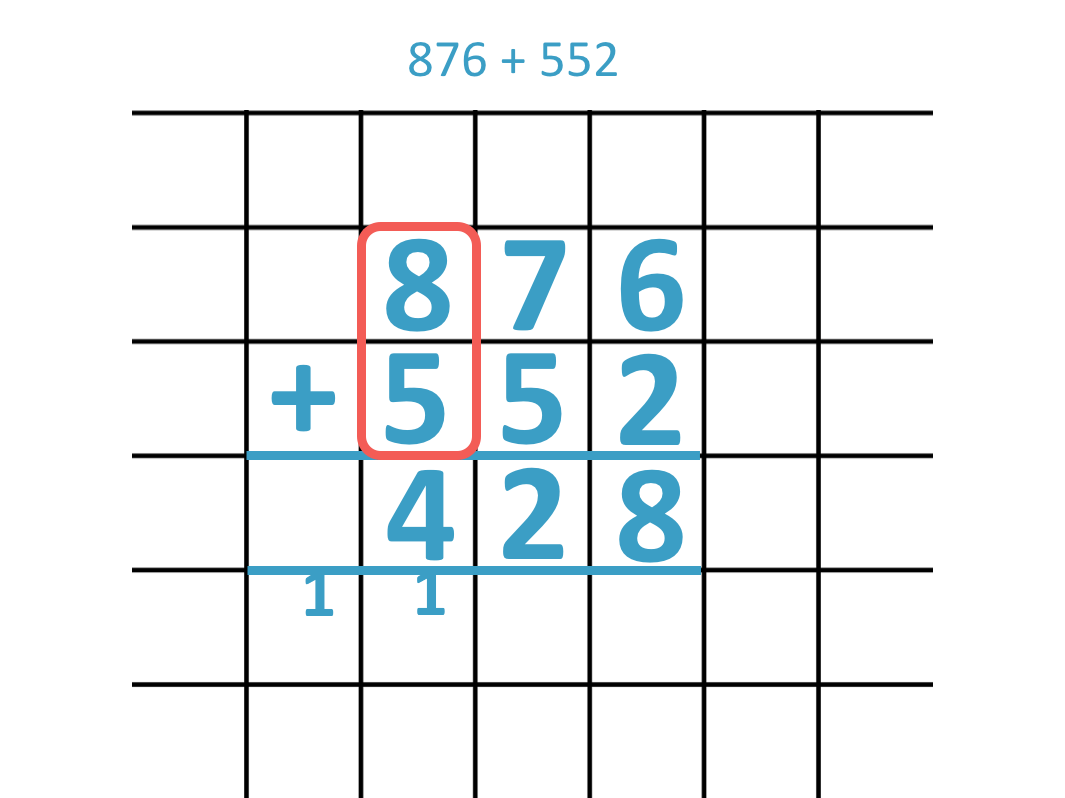We have no other digits in the thousands column and so, we write the '1' in the thousands column.876 + 552 = 1428.Now try our lesson on Column Subtraction without Borrowing / Regrouping where we learn how to solve subtraction problems using the column subtraction method.